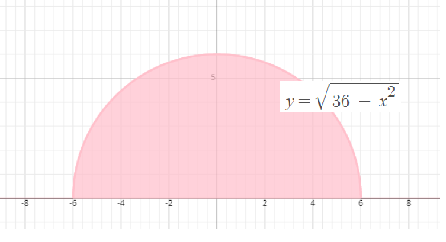# Evaluate the integral below by interpreting it in terms of areas. In other words, draw a picture...

## Question:

Evaluate the integral below by interpreting it in terms of areas. In other words, draw a picture of the region the integral represents, and find the area using geometry.

{eq}\int_{-6}^{6} \sqrt{36 - x^2}\ dx {/eq}

## Area Estimation:

It is possible to find the area under a curve between two points by making the definite integral between the two points. To find the area between x= a and x= b and y= f(x), integrate y= f(x) between a and b limits. Areas below the x-axis will be negative, and areas above the x-axis will be positive.

Given that {eq}\int_{-6}^{6} \sqrt{36 - x^2}\ dx {/eq}

The graph of the above function as followsApply trigonometric substitution, we get

{eq}x=6\sin \left(u\right), dx=6\cos \left(u\right)du\\ x=6\rightarrow dx=\frac{\pi }{2}\\ x=-6\rightarrow dx=-\frac{\pi }{2}\\ {/eq}

Apply these values, we get

{eq}\int_{-6}^{6} \sqrt{36 - x^2}\ dx=\int _{-\frac{\pi }{2}}^{\frac{\pi }{2}}36\cos ^2\left(u\right)du\\ =36\cdot \int _{-\frac{\pi }{2}}^{\frac{\pi }{2}}\frac{1+\cos \left(2u\right)}{2}du\\ =36\cdot \frac{1}{2}\left(\int _{-\frac{\pi }{2}}^{\frac{\pi }{2}}1du+\int _{-\frac{\pi }{2}}^{\frac{\pi }{2}}\cos \left(2u\right)du\right)\\ =\left[u\right]^{\frac{\pi }{2}}_{-\frac{\pi }{2}}+\frac{1}{2}\left[\sin \left(2u\right)\right]^{\pi }_{-\pi }\\ =36\cdot \frac{1}{2}\left(\pi +0\right)\\ =18\pi {/eq}